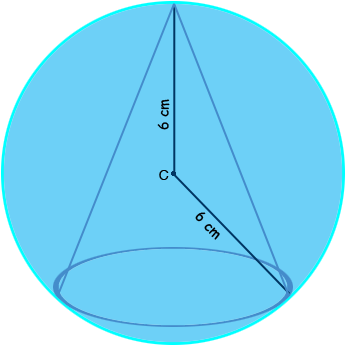SEARCH HOMEMath Central Quandaries & QueriesQuestion from Alfredo, a student: What is the altitude of the largest circular cone that may be cut out from a sphere of radius 6 cm?Hi Alfredo,

The volume $V$ of a circular cone of base radius $r$ and height $h$ is given by

$V = \frac13 \pi \; r^2 h.$What is the slant height of the cone?

PennyMath Central is supported by the University of Regina and the Imperial Oil Foundation.